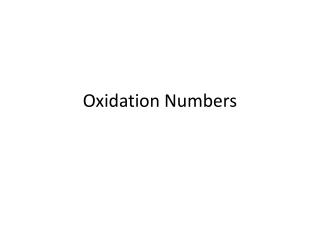DownloadDownload PresentationOxidation Numbers

# Oxidation Numbers

Télécharger la présentation## Oxidation Numbers

- - - - - - - - - - - - - - - - - - - - - - - - - - - E N D - - - - - - - - - - - - - - - - - - - - - - - - - - -
##### Presentation Transcript

1. Oxidation Numbers

2. Oxidation Number • A number equal to the charge that an atom would have if no electrons were shared but instead were possessed by the atom with the greatest electronegativity

3. Assigning Oxidations Numbers 1. A pure element: zero. 2. An element in a monatomic ion equals the charge on the ion. 3. Hydrogen in compounds is +1, except in metal hydrides, where it is −1. 4. Oxygen in compounds is usually −2, but there are exceptions. [ex. peroxides, such as H2O2(ℓ), it is -1]

4. 5. In molecular compounds without hydrogen or oxygen, the more electronegative element is assigned an oxidation number equal to its ionic charge. 6. Sum of oxidation numbers in a neutral compound is zero. 7. Sum of oxidation numbers in a polyatomic ion equals the charge on the ion.

5. Ex. Assign an oxidation number to SiBr4(ℓ) • {Rule 5} Bromine has a higher electronegativity, the bromine ion is assigned a charge of 1-. • {Rule 6} 1 Si atom + 4 Br atoms = 0 x + 4(-1) = 0 x - 4 = 0 x = +4 The oxidation number of Si is +4 and Br is -1.

6. Ex. Assign an oxidation number to HClO4(aq) • {Rule 3} The oxidation number of oxygen is -2 and the oxidation number of hydrogen is +1. • {Rule 6} 1 H atom + 1 Cl atom + 4 O atoms = 0 1(+1) + 1(x) + 4(-2) = 0 1 + x - 8 = 0 x = +7 The oxidation number of H is +1, Clis +7, and O is -2.

7. Ex. Assign an oxidation number to Cr2O72−(aq) • {Rule 4} The oxidation number of oxygen is -2. • {Rule 7} The sum of oxidation numbers is -2. 2 Cr atoms + 7 O atoms = -2 2x + 7(-2) = -2 2x - 14 = -2 2x = 12 x = +6 The oxidation number of O is -2 and Cr is +6.

8. Assigning Non-integer Oxidation Numbers • When applying the rules, you will discover that some oxidation numbers do not appear to be integers. • This is not an error • Indicates multiple oxidation numbers for one type of element [ie. Iron (II) and iron (III)]

9. Applying Oxidation Numbers to Redox Reactions • You can use oxidation numbers to identify redox reactions and to identify the oxidizing and reducing agents. • A redox reaction is a reaction in which the oxidation numbers of at least two atoms change. • Oxidation is an increase in oxidation number. • Reduction is a decrease in oxidation number.

10. Determine whether the following is a redox reaction. If so, identify the oxidizing agent and the reducing agent. CH4(g) + Cl2(g) → CH3Cl(g) + HCl(g)

11. Determine whether the following is a redox reaction. If so, identify the oxidizing agent and the reducing agent. CaCO3(s) + 2HCl(aq) → CaCl2(aq) + H2O(ℓ) + CO2(g)

12. Determine whether the following is a redox reaction. If so, identify the oxidizing agent and the reducing agent. 3HNO2(aq) → HNO3(aq) + 2NO(g) + H2O(ℓ)

13. Balancing Using Oxidation Numbers Step 1 Write an unbalanced equation. Step 2 Assign oxidation numbers and determine if it is a redox reaction. Step 3 If a redox reaction, identify the atom or atoms that increase and decrease oxidation number. Step 4 Determine the numerical values for change in oxidation. Step 5 Determine the lowest common multiple for change in oxidation.

14. Step 6 Apply the numbers determined in step 5. Step 7 **Non acid/base** Balance all elements by inspection. Step 8 **acid** Balance any oxygen atoms by adding water molecules, all other by inspection. Then balance the hydrogen atoms by adding hydrogen ions. Step 9 **base** Complete step 8, and then add hydroxide ions to both sides to neutralize the hydrogen ions. Cancel any water molecules that are on both sides of the equation.

15. The dichromate ion reacts with ethanol in an acidic solution to produce the chromium(III) ion and carbon dioxide. Write a balanced equation for the reaction using the oxidation number method. Cr2O72-(aq) + C2H5OH(aq) → Cr3+(aq) + CO2(g) 16H+(aq) + 2Cr2O72−(aq) + C2H5OH(aq) → 4Cr3+(aq) + 2CO2(g) + 11H2O(ℓ)